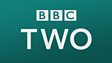The inverse calculation is used to check how many lumps of sugar have been added to a cup of tea. Two cartoon characters are drinking tea. One puts ten lumps of sugar from a bowl into his cup. He checks how many he has added by counting how many are left in the bowl. He then adds a further 21 lumps to his cup, again checking the number he has put in his tea by counting the lumps left in the bowl.This clip is from:
Numbertime, Addition And Subtraction: Plus and Minus
First broadcast:
3 December 2001

After watching the clip, the inverse nature of addition and subtraction can be illustrated by getting children to walk along a number track from a given starting point. For example, start on three and add seven (by jumping seven places to the left) and reaching ten. Then turn round and subtract seven. Children should ‘realise’ that they are back where they started which is what is meant by an inverse operation. By asking children to find the ‘odd one out’ of three calculations (one of which is incorrect) children could be encouraged to use addition to check subtraction eg 'Which is the odd one out? 43 – 17 = 26, 54 - 36 = 18 or 23 - 14 = 8?' (Answer: 23 -14 is the odd one out because 8 + 14 = 22).